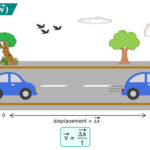Home / Physics / Heat Transfer

# Heat Transfer

Heat transfer refers to the phenomenon in which heat or thermal energy is transferred from one region to another. The movement of atoms, ions, and molecules is responsible for carrying the energy. Heat is transferred from a high-temperature region to a low-temperature region. It can also occur between two thermodynamic systems at different temperatures, resulting in changes in internal energies.

## Types of Heat Transfer

Three types of heat transfer occur in nature – conduction, convection, and thermal radiation.

### 1. Conduction

Heat is transferred between two atoms or molecules in direct contact. The transfer occurs when agitated molecules at high temperatures strike slower molecules at low temperatures, resulting in collisions. Heat transfer occurs through vibrations if the atoms are fixed in a lattice. Conduction takes place in solid, liquid, and gas. For example, when we heat one end of a metal rod, the energy quickly transmits to the other.

Equation

According to Fourier’s Law for heat conduction, the heat transfer rate is proportional to the negative temperature gradient and the area at right angles to the gradient through which heat flows. Mathematically, the equation is given by

$Q = – KA \frac{\Delta T}{\Delta x}$

Where

Q: Heat transfer per unit time

K: Thermal conductivity

A: Cross-sectional area of the conductor

$$\frac{\Delta T}{\Delta x}$$: Temperature gradient

### 2. Convection

Energy is transferred through a medium like liquid or gas. Molecules carry the energy due to the motion of the fluid. For example, when we boil water in a pan, the molecules at the bottom get heated first and carry the energy to the top. In nature, we experience convection currents due to hot air close to the ground that expands, rises, and cools down, resulting in a circular movement.

Energy is transferred without any contact between the atoms or molecules. Energy in the form of electromagnetic radiation is emitted by a heated body and absorbed by another. A medium is not required for energy to travel. Electromagnetic waves carry thermal energy. For example, Sun emits heat through radiation, and we feel its warmth.

Equation

The intensity of radiation depends on the temperature and the surface characteristics. The radiant heat energy emitted per second per unit area by a surface is proportional to the fourth power of its absolute temperature. It is the basis of Stefan-Boltzmann law, which is represented as follows:

$\frac{P}{A} = \sigma T^4$

Where

P: Radiant heat energy emitted per second

A: Surface area of the object emitting heat

σ: Stephan-Boltzmann constant

T: Temperature of the object

Article was last reviewed on Monday, January 2, 2023

### Related articlesLaminar FlowRadius of GyrationNewton’s Law of CoolingVelocity